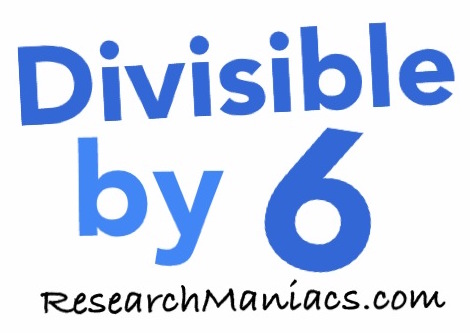Is 246 divisible by 6?

 The question we are solving here is: If we divide 246 by 6, will we get a whole number with no remainder? In other words, is 246 divisible by 6? For 246 to be divisible by 6, both of the following two criteria must be met: First, 246 has to be an even number and second, the sum of its digits must be divisible by 3. Looking at 246, you see that 246 is an even number, which means that the first criteria is met. The sum of the digits in 246 is 12, which is divisible by 3. Therefore, the second criteria is met. Thus, 246 is divisible by 6 and the answer to the question "Is 246 divisible by 6?" is YES You may also be interested to know that if you divide 246 by 6, you get 41. Is 247 divisible by 6? Can you do it on your own now? If not, go here for the next number on our list.Divisible by 6? Do you need to check another number? Enter a number below to see if it is divisible by 6.